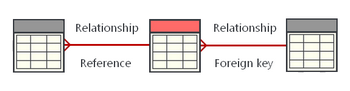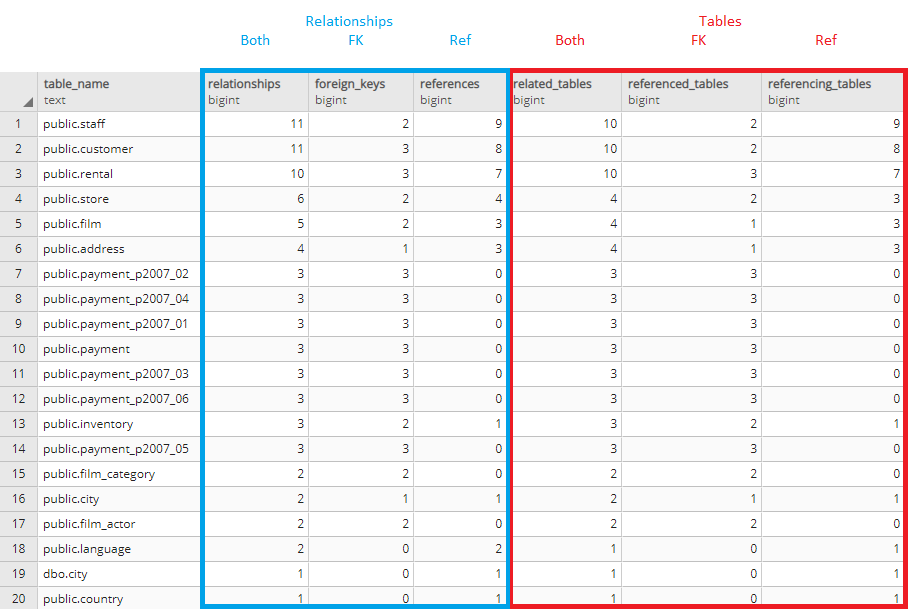## List tables with most relationships in PostgreSQL database

Article for:

Query shows tables with their relationships statistics.## Query

``````select relations.table_name as table_name,
count(relations.table_name) as relationships,
count(relations.referenced_tables) as foreign_keys,
count(relations.referencing_tables) as references,
count(distinct related_table) as related_tables,
count(distinct relations.referenced_tables) as referenced_tables,
count(distinct relations.referencing_tables) as referencing_tables
from(
select pk_tco.table_schema || '.' || pk_tco.table_name as table_name,
fk_tco.table_schema || '.' || fk_tco.table_name as related_table,
fk_tco.table_name as referencing_tables,
null::varchar(100) as referenced_tables
from information_schema.referential_constraints rco
join information_schema.table_constraints fk_tco
on rco.constraint_name = fk_tco.constraint_name
and rco.constraint_schema = fk_tco.table_schema
join information_schema.table_constraints pk_tco
on rco.unique_constraint_name = pk_tco.constraint_name
and rco.unique_constraint_schema = pk_tco.table_schema
union all
select fk_tco.table_schema || '.' || fk_tco.table_name as table_name,
pk_tco.table_schema || '.' || pk_tco.table_name as related_table,
null as referencing_tables,
pk_tco.table_name as referenced_tables
from information_schema.referential_constraints rco
join information_schema.table_constraints fk_tco
on rco.constraint_name = fk_tco.constraint_name
and rco.constraint_schema = fk_tco.table_schema
join information_schema.table_constraints pk_tco
on rco.unique_constraint_name = pk_tco.constraint_name
and rco.unique_constraint_schema = pk_tco.table_schema
) relations
group by table_name
order by relationships desc;
``````

## Columns

• table_name - schema name and table name
• relationships - number of table relationships
• foreign_keys - number of foreign keys in a table
• references - number of foreign keys that are refering to this table
• related_tables - number of related tables
• referenced_tables - number of different tables referenced with FKs (multiple FK's can refers to one table, so number of foreign keys might be diffrent than number of referenced tables)
• referencing_tables - number of diffrent tables that are refering to this table (similar note like as above)

## Rows

• One row: one table in database
• Scope of rows: all tables with any relationship
• Ordered by number of relationships descending

## Sample results0